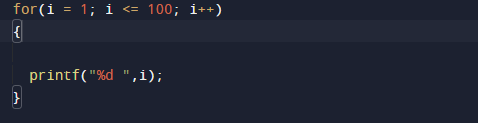C Program to print from 1 to 100 numbers

In this tutorial you will learn about the C Program to print from 1 to 100 numbers and its application with practical example.

In this tutorial, we will learn to create a C program that  will print 1-100 number  using C programming.

Prerequisites

Before starting with this tutorial we assume that you are best aware of the following C programming topics:

• C Operators.
• Basic input/output.
• Basic C Programming.
• Looping statements.
• For loop.

Program to print from 1 to 100 numbers.

In the example, we are going to write a Program to Print 1 to 100 number  with using  for Loops.Let’s see the C code to print 1 to 100 numbers using for  loop.

Program to demonstrate series 1-100.

In this program we will print a series from 1-100 using for loop.
Firstly we declare required header file and variable and also initiates required values in variable. Next we print the series  from  1-100 using loop statement.

In this above program , we are using for loop to print numbers from 1 to 100.

OutputIn our  program, we have first declared  required  variables in the program.

• i= for iteration from 1-100.

firstly we have to create a variable named i

Within the program, we used the for loop Statement to check the value of i is less than or equal to 100(i<=100). If the condition is true ,then statements inside the loop will be executedWhile the condition is true the print statement from the loop body is executed.which will help to print the series from 1 to 100 .

In this tutorial we have learn about the C Program to print from 1 to 100 numbers and its application with practical example. I hope you will like this tutorial.